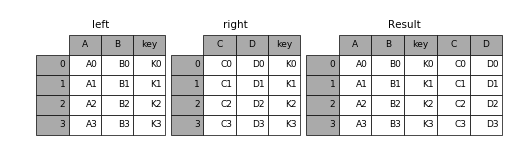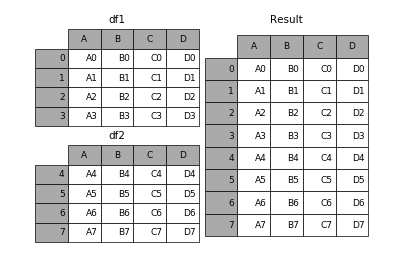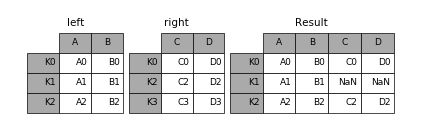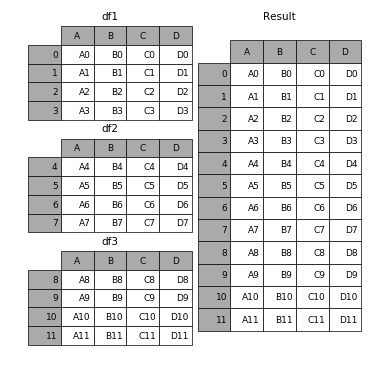• pandas提供了各种工具，在连接/合并类型操作的情况下，可以方便地序列、数据帧和面板对象与各种索引集逻辑和关系代数功能组合在一起。 1. merge pd.merge(left, right, how='inner', on=None, left_on=None, right...
pandas提供了各种工具，在连接/合并类型操作的情况下，可以方便地将序列、数据帧和面板对象与各种索引集逻辑和关系代数功能组合在一起。
1. merge
pd.merge(left, right, how='inner', on=None, left_on=None, right_on=None,
left_index=False, right_index=False, sort=True,
suffixes=('_x', '_y'), copy=True, indicator=False)

left︰ 对象
right︰ 另一个对象
on︰ 要加入的列 （名称）。必须在左、 右综合对象中找到。如果不能通过 left_index 和 right_index 是假，将推断 DataFrames 中的列的交叉点为连接键
left_on︰ 从左边的综合使用作为键列。可以是列名或数组的长度等于长度综合
right_on︰ 从正确的综合，以用作键列。可以是列名或数组的长度等于长度综合
left_index︰ 如果为 True，则使用索引 （行标签） 从左综合作为其联接键。在与多重 （层次） 的综合，级别数必须匹配联接键从右综合的数目
right_index︰ 相同用法作为正确综合 left_index
how︰ 之一 ‘左’，‘右’，‘外在’、 ‘内部’。默认为内部。每个方法的更详细说明请参阅︰
sort︰ 综合通过联接键按字典顺序对结果进行排序。默认值为 True，设置为 False将提高性能极大地在许多情况下
suffixes︰ 字符串后缀并不适用于重叠列的元组。默认值为 (’_x’，’_y’)。
copy︰ 即使重新索引是不必要总是从传递的综合对象，复制的数据 （默认值True）。在许多情况下不能避免，但可能会提高性能 / 内存使用情况。可以避免复制上述案件有些病理但尽管如此提供此选项。
indicator︰ 将列添加到输出综合呼吁 _merge 与信息源的每一行。_merge 是绝对类型，并对观测其合并键只出现在 ‘左’ 的综合，观测其合并键只会出现在 ‘正确’ 的综合，和两个如果观察合并关键发现在两个 right_only left_only 的值。
展开全文python
• os.listdir(filepath) features = pd.DataFrame() for file in allfile: feature = pd.read_csv(filepath + file, encoding='ANSI') features = features.append(feature) features.to_csv(outpath, index=False)
方法1：
import pandas as pd
import os

output_file = 'D:/PycharmProjects/DataProcess/MeteData/out/AllYear_'
input_path = 'D:/PycharmProjects/DataProcess/MeteData/out/v10/'

files = os.listdir(input_path)
all_data_frames = []
for file in files:
all_data_frames.append(data_frame)

data_frame_concat = pd.concat(all_data_frames, axis=0, ignore_index=True)
data_frame_concat.to_csv(output_file+file, index=False)
方法2：
import pandas as pd
import os

filepath = 'D:\\PycharmProjects\\DataProcess\\Check_MatchResult\\'
outpath = 'C:\\Users\\user\\Desktop\\testout.csv'

allfile = os.listdir(filepath)
features = pd.DataFrame()
for file in allfile:
feature = pd.read_csv(filepath + file, encoding='ANSI')
features = features.append(feature)

features.to_csv(outpath, index=False)

展开全文python csv
• python 把几个DataFrame合并成一个DataFrame——merge,append,join,conca
 python  把几个DataFrame合并成一个DataFrame——merge,append,join,conca

pandas provides various facilities for easily combining together Series, DataFrame, and Panel objects with various kinds of set logic for the indexes and relational algebra functionality in the case of join / merge-type operations.

1、merge

pd.merge(left, right, how='inner', on=None, left_on=None, right_on=None,
left_index=False, right_index=False, sort=True,
suffixes=('_x', '_y'), copy=True, indicator=False)

left
︰

对象

right
︰

另一个
对象

on︰

要
加入
的
列

（名称）
。
必须
在
左
、

右
综合
对象
中
找到
。
如果
不
能
通过

left_index

和

right_index

是
假
，
将
推断

DataFrames

中
的
列
的
交叉点
为
连接
键

left_on
︰

从
左边
的
综合
使用
作为
键
列
。
可以
是
列名
或
数组
的
长度
等于
长度
综合

right_on
︰

从
正确
的
综合
，
以
用作
键
列
。
可以
是
列名
或
数组
的
长度
等于
长度
综合

left_index
︰
如果为
True
，
则
使用
索引

（行
标签）

从
左
综合
作为
其
联接
键。
在
与
多重

（层次）
的
综合
，
级别
数
必须
匹配
联接
键
从
右
综合
的
数目

right_index
︰

相同
用法
作为
正确
综合

left_index

how︰

之一

'左'，
'右'，
'外在'、

'内部'。
默认
为
内部
。
每个
方法
的
更
详细
说明
请
参阅
︰

sort︰

综合
通过
联接
键
按
字典
顺序
对
结果
进行排序
。
默认值
为

True
，
设置
为

False

将
提高
性能
极大地
在
许多
情况下

suffixes︰

字符串
后缀
并
不适
用于
重叠
列
的
元组
。
默认值
为

('_x'，
'_y')。

copy︰

即使
重新索引
是
不
必要
总是
从
传递
的
综合
对象
，
复制
的
数据

（默认值

True）
。
在
许多
情况下
不能
避免
，
但
可能会
提高
性能

/

内存
使用情况
。
可以
避免
复制
上述
案件
有些
病理
但
尽管如此
提供
此
选项
。

indicator︰
将
列
添加
到
输出
综合
呼吁

_merge

与
信息
源
的
每
一行
。
_merge

是
绝对类型
，
并
对
观测
其
合并
键
只
出现
在

'左'
的
综合
，
观测
其
合并
键
只
会出现
在

'正确'
的
综合
，
和
两个
如果
观察
合并
关键
发现
在
两个

right_only

left_only
的
值
。

1.result = pd.merge(left, right, on='key')2.result = pd.merge(left, right, on=['key1', 'key2'])

3.result = pd.merge(left, right, how='left', on=['key1', 'key2'])4.result = pd.merge(left, right, how='right', on=['key1', 'key2'])5.result = pd.merge(left, right, how='outer', on=['key1', 'key2'])2、append

1.result = df1.append(df2)2.result = df1.append(df4)

3.result = df1.append([df2, df3])

4.result = df1.append(df4, ignore_index=True)4、join

left.join(right, on=key_or_keys)
pd.merge(left, right, left_on=key_or_keys, right_index=True,
how='left', sort=False)
1.result = left.join(right, on='key')2.result = left.join(right, on=['key1', 'key2'])3.result = left.join(right, on=['key1', 'key2'], how='inner')4、concat

pd.concat(objs, axis=0, join='outer', join_axes=None, ignore_index=False,
keys=None, levels=None, names=None, verify_integrity=False,
copy=True)

objs︰

一个
序列
或
系列
、

综合
或
面板
对象
的
映射
。
如果
字典
中
传递
，
将
作为
键
参数
，
使用
排序
的
键
，
除非
它
传递
，
在
这
种情况下
的
值
将
会
选择

（见
下文）。
任何
没有任何
反对
将
默默地
被
丢弃
，
除非
他们
都
没有
在
这
种情况下
将
引发

ValueError
。

axis:

{0，
1，
...}，
默认值
为 0。
要
连接
沿
轴
。

join
:

{'内部'、

'外'}，
默认

'外'。
如何
处理
其他

axis(es)

上
的
索引
。
联盟
内
、

外
的
交叉口
。

ignore_index
︰

布尔值
、

默认

False
。
如果
为
True
，
则
不要
串联
轴
上
使用
的
索引
值
。
由此产生
的
轴
将
标记

0，
...
，
n
-
1。
这
是
有用
的
如果
你
串联
串联
轴
没有
有意义
的
索引
信息
的
对象
。
请注意
在
联接
中
仍然
受到尊重
的
其他
轴
上
的
索引
值
。

join_axes
︰

索引
对象
的
列表
。
具体
的
指标
，
用于
其他

n
-
1

轴
而不是
执行
内部/外部
设置
逻辑
。

keys
︰

序列
，
默认
为
无
。
构建
分层
索引
使用
通过
的
键
作为
最
外面
的
级别
。
如果
多个
级别
获得通过
，
应
包含
元组
。

levels
︰

列表
的
序列
，
默认
为
无
。
具体
水平

（唯一
值）

用于
构建
多重
。
否则
，
他们
将
推断
钥匙
。

names
︰

列表中
，
默认
为
无
。
由此产生
的
分层
索引
中
的
级
的
名称
。

verify_integrity
︰

布尔值
、

默认

False
。
检查
是否
新
的
串联
的
轴
包含
重复项
。
这
可以
是
相对于
实际
数据
串联
非常
昂贵
。

副本
︰

布尔值
、

默认

True
。
如果
为
False
，
请
不要
，
不必要地
复制
数据
。

1.frames = [df1, df2, df3]

2.result = pd.concat(frames)3.result = pd.concat(frames, keys=['x', 'y', 'z'])

4.result.ix['y']
A   B   C   D
4  A4  B4  C4  D4
5  A5  B5  C5  D5
6  A6  B6  C6  D6
7  A7  B7  C7  D7
5.result = pd.concat([df1, df4], axis=1)

6.result = pd.concat([df1, df4], axis=1, join='inner')

7.result = pd.concat([df1, df4], axis=1, join_axes=[df1.index])

8.result = pd.concat([df1, df4], ignore_index=True)连接：
http://pandas.pydata.org/pandas-docs/stable/merging.html


展开全文python merge append
• # -*- coding: utf-8 -*- """ Created on Mon Jan 20 13:02:27 2020 @author: twx253 """ import xlrd ...from pandas import DataFrame from openpyxl import load_workbook excel_name = 'C:...
# -*- coding: utf-8 -*- """ Created on Mon Jan 20 13:02:27 2020
@author: twx253 """
import xlrd import pandas as pd from  pandas import DataFrame from openpyxl import load_workbook
excel_name = 'C:/Users/twx253/Desktop/many_sheets.xlsx'   wb = xlrd.open_workbook(excel_name) sheets = wb.sheet_names() # 获取workbook中所有的表格 # 循环遍历所有sheet alldata = DataFrame() for i in range(len(sheets)):     df = pd.read_excel(excel_name, sheet_name=i, index=False, encoding='utf8')     print(df.shape)     alldata = alldata.append(df)      #指定合并后的数据放在哪个excel里 writer = pd.ExcelWriter('C:/Users/twx253/Desktop/many_sheets.xlsx',engine='openpyxl')  book = load_workbook(writer.path) writer.book = book
#指定合并后的数据放在哪个sheet里 alldata.to_excel(excel_writer=writer,sheet_name="ALLDATA",index=False) writer.save() writer.close() print('处理完成！')
# ============================================================================= # 现在需要将dataframe1,dataframe2和dataframe3中的数据存放到result.xlsx中的三个不同的sheet中, # sheet的名字分别为dataframe1,dataframe2,datafame3. # ============================================================================= writer = pd.ExcelWriter('result.xlsx') for i in ['dataframe1','dataframe2','dataframe3']:        eval(i).to_excel(excel_writer=writer,sheet_name=i,index=False) writer.save() writer.close()  
展开全文• Is there any nicer way to prefix or rename all or multiple columns at the same time of a given SparkSQL DataFrame than calling multiple times dataFrame.withColumnRenamed()?An example would be if I wan...
•python
• 一、简介 python中pandas的DataFrame是数据分析中常用的数据结构之一，通常从外部文件中导入的文件都是存储为DataFrame格式的，因此掌握DataFrame的相关操作...二、DataFrame合并 其实可以python的DataFra...
• import pandas as pd df = pd.DataFrame({'uid': [1, 2, 3, 4], 'B': [1, 2, 3, 4], 'C': [4,5,6,7]}) df2=pd.DataFrame({'uid':[1,1,2,2], 'hello':['...
• Python中如何将多个dataframe表连接、合并成一个dataframe详解示例--pandas中merge、join、concat、append的区别、用法梳理 我们在对Pandas中的DataFrame对象进行，表的连接、合并的时候，好像merge可以join也可以...
• ## dataframe合并

千次阅读 2019-01-18 00:09:20
pandas提供了一个类似于关系数据库的连接(join)操作的方法merage,可以根据一个或多个不同DataFrame中的行连接起来 语法如下： merge(left, right, how='inner', on=None, left_on=None, right_on=None,  left_...
• python:pandas 合并多个DataFrame python 把几个DataFrame合并成一个DataFrame——merge,append,join,concat 1、mergepd.merge(left,right,how=‘inner’,on=None,left_on=None,right_on=None,left_index=False,...pandas python
• 将多个数据表，以时间为索引，并选取需要分析的列进行合并。在本文中，拿到的数据是同一时期的不同设备的特征数据，需要提取对应的列进行时间合并，在合并过程中存在以下问题： 1、时间索引存在重复，需要删除重复项...python
• 例如c是这：   age weight high 0 15 100 167 1 18 120 178 d是这：   age weight high 3 24 134 175 ...
• 转载... python 把几个DataFrame合并成一个DataFrame——merge,append,join,concat pandas provides various facilities for easily combining together Series, DataFrame, and Panel o...
• 解决问题：一个循环会产生多个单列的Dataframe，我想一个循环下来，用一个Dataframe把这些Dataframe连接起来。 需要用到的函数是pd.contcat， df2 = pd.DataFrame() cell_list = [1,2,4,5,6,8] for cell_num ...
• #两个dataframe合并 #merge并保留不匹配的数据为NA值 #多个dataframe数据的合并 #dplyr包的join功能 #仿真数据 data1 <- data.frame(id = 1:6, # Create first example data frame ...r语言 python 人工智能 数据挖掘 机器学习
• 今天小编就为大家分享一篇Pandas 合并多个Dataframe(merge,concat)的方法，具有很好的参考价值，希望对大家有所帮助。一起跟随小编过来看看吧
• video_df_list=[] video_df_list.append(video_df_1) video_df_list.append(video_df_2) video_df_list.append(video_df_3) video_df_list.append(video_df_4) #结果合并在一块 all_video_df=pd...
• import pandas as pdimport glob def GetFile(fname): df = pd.read_excel(fname) # 记录文件名 df['File'] = fname # 设置 "File" 列为索引 return df.set_index(['File']) df_list = [GetFile...
• 这里介绍一下多个相同格式的excel合并的处理。如果在excel文件表比较少时，手工合并还可以应付，当几十个几百个甚至更多的表时，手工合并效率会非常低，于是让计算机自动合并处理，帮助我们完成工作就显得非常有必.....
• 要实现这功能，可能有多种方法，我在这里记录下一比较方便的方法： import pandas as pd writer = pd.ExcelWriter('test.xlsx') data1.to_excel(writer,sheet_name='sheet1') data2.to_excel(writer,sheet_name...
• 多个series合并dataframe s1 = data[“PicFixationDuration”].value_counts() s2 = data[“WordFixationDuration”].value_counts() result = pd.DataFrame(list(zip(s1,s2))) print(result) s1 和 s2 是两个...pandas series python
• 1、 两个dataframe 外连接 df3= df.merge(df2,on =['datekey','user_id','is_pay'],how = 'outer') ...2、多个dataframe外连接合并 以txt格式存储的多个本地文件为例（1018.txt，1019.txt），文件名分别为：......